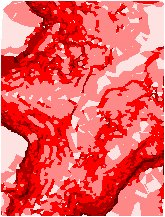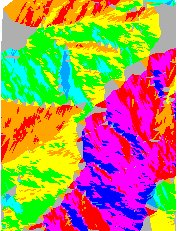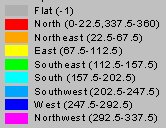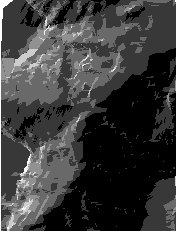Go to ET GeoWizards 12.x User Guide

ESRI TIN to PolygonZ

Go to ToolBox Implementation

Converts an ESRI TIN to PolygonZ feature class. Calculates several  characteristics  for each triangle.

• Minimum elevation
• Maximum elevation
• Mean elevation
• Slope - identifies the slope, or maximum rate of elevation change for each triangle
• Aspect - the values of the output field represent the compass direction of the aspect; 0 is true north, a 90 degree aspect is to the east etc. For  flat triangles (slope = 0) the value of -1 is assigned for the aspect
• Hill Shade- computes the brightness of each triangle based on a light source location.

Inputs:

• A  TIN in ESRI TIN format
• Characteristics to be calculated
• Parameters for Hill Shade ( if Hill Shade option is selected )
• azimuth - the azimuth angle of the light source. The azimuth is expressed in positive degrees from 0 to 360, measured clockwise from the north. The default is 315 degrees.
• altitude - the altitude angle of the light source above the horizon. The altitude is expressed in positive degrees, with 0 degrees at the horizon and 90 degrees directly overhead. The default is 45 degrees.

Outputs:

• New PolygonZ feature class. Several fields are added to the attribute table, depending on the options selected
• [ET_ElMin] - minimum elevation values for each triangle
• [ET_ElMax] - maximum elevation values for each triangle
• [ET_ElMean] - mean elevation values for each triangle
• [ET_Slope] - the slope of each triangle
• [ET_Aspect] - the aspect of each triangle
• [ET_Hill] - a Hill Shade value for each triangle depending on the input values for Azimuth and Altitude of the light source

Notes:

• A flat triangle ( Slope = 0 ) will have an Aspect of -1

Example: TIN ( PolygonZ ) classified by

 MIN Elevation SlopeAspect Hill ShadeCommand line syntax

ET_GPEsriTINToPolygonZ <input_TIN> <out_feature_class> <Light_Azimuth><Light_Altitude>

Parameters

Expression Explanation
<input_TIN> A  TIN layer or dataset
<out_feature class> A String - the full name of the output feature class (A feature class with the same full name should not exist)
{Light_Azimuth} A Double representing the azimuth angle of the light source. The azimuth is expressed in positive degrees from 0 to 360, measured clockwise from the north. The default is 315 degrees.
{Light_Altitude} A Double representing the altitude angle of the light source above the horizon. The altitude is expressed in positive degrees, with 0 degrees at the horizon and 90 degrees directly overhead. The default is 45 degrees.

Scripting syntax

ET_GPEsriTINToPolygonZ (input_TIN out_feature_class Light_Azimuth Light_Altitude)

See the explanations above:
<> - required parameter
{} - optional parameter